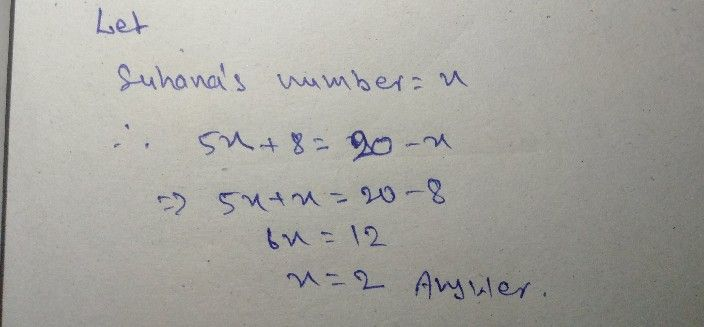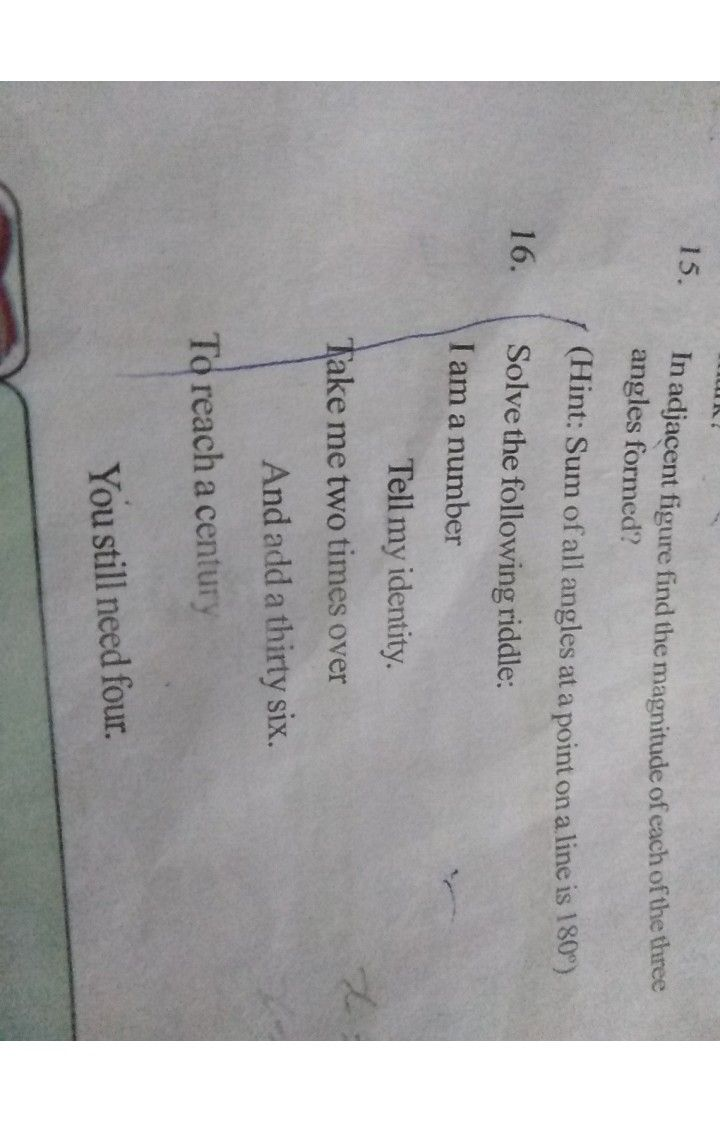Symbol
ProblemSuhana said, $1$ $\bar{x} 18=26-x$ "multiplying my number by $5$ and adding $8$ to it same answer as subtracting my number from $20^{°}$ Find Suhana's numbers. teoch by a stu Cst $naFk1s$ 8 In adjacent figure find the magnitude of each of the three angles fo